﻿ EN600变频器助力冷弯型钢机组-EN600变频器-技术文章-中国工控网

# EN600变频器助力冷弯型钢机组

### 0人气：42018年10月23日一座跨越伶仃洋，东接香港，西接广东珠海和澳门，总长约55公里，由粤港澳三地首次合作共建的超大型跨海大桥正式开通。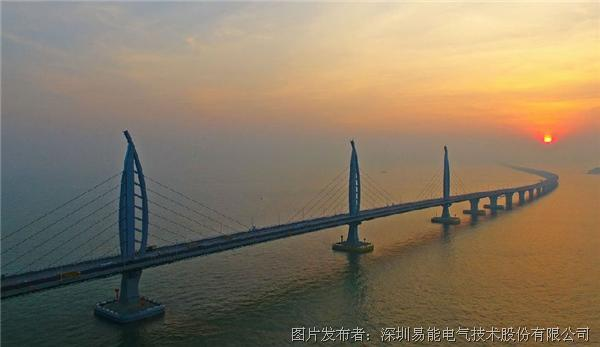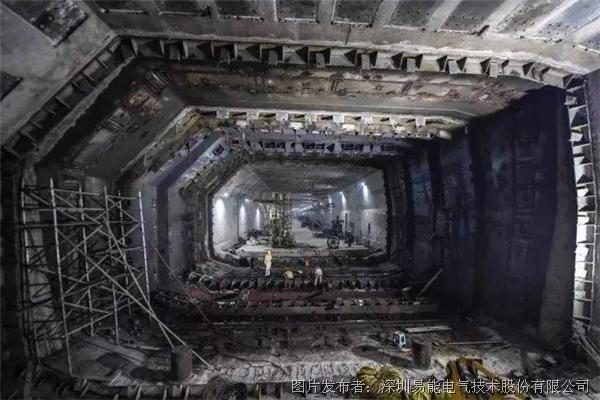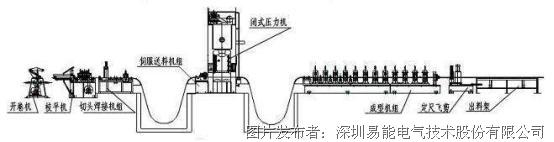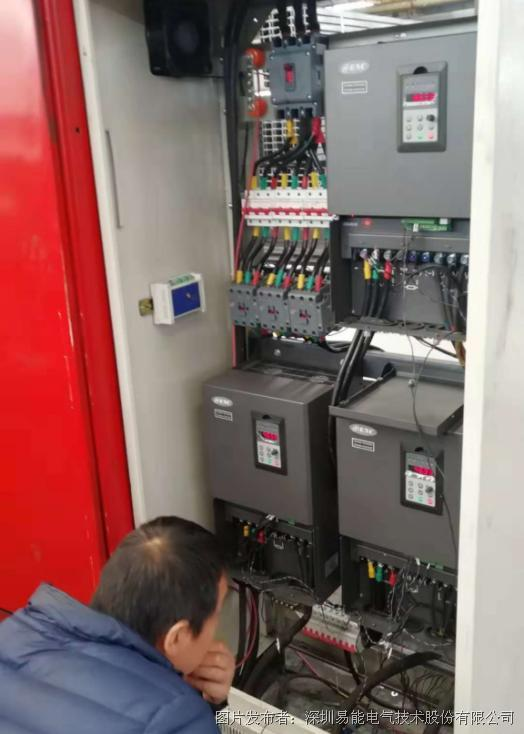1）自整定设置，F00.24=1，F15.01=37，F15.02=380，F15.03=68，F15.04=50，F15.05=1440，F15.06=2，F15.19=1.设置好后按RUN键进行电机参数静止自整定

2）主机参数：F00.00=2，F01.00=1，F01.15=1，F02.11=1，F09.35=2，F09.36=17,F19.43=100

3）从机参数：F00.00=2，F01.15=1，F09.35=2，F09.36=16，F14.00=1，F14.13=002，F14.16=1，F14.17=1，F19.43=100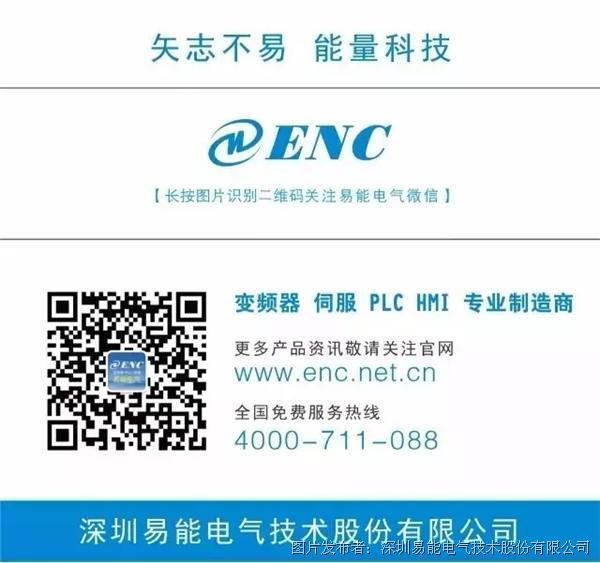评价： 一般